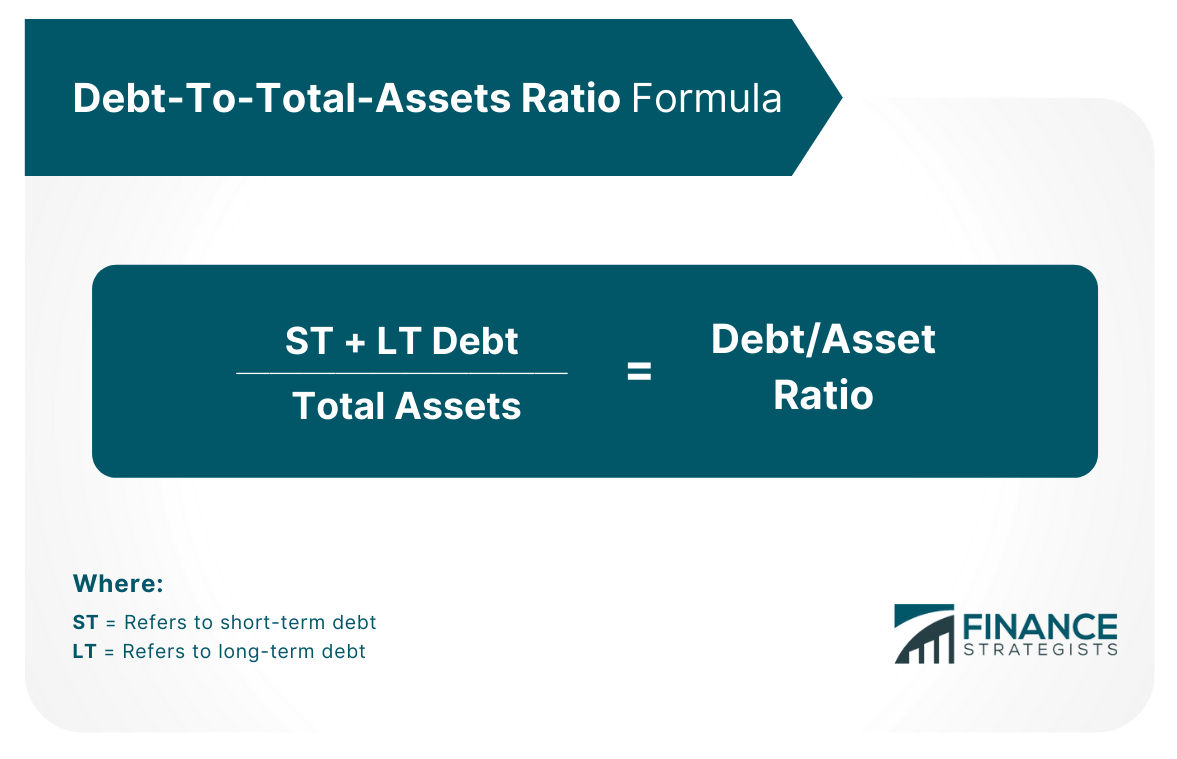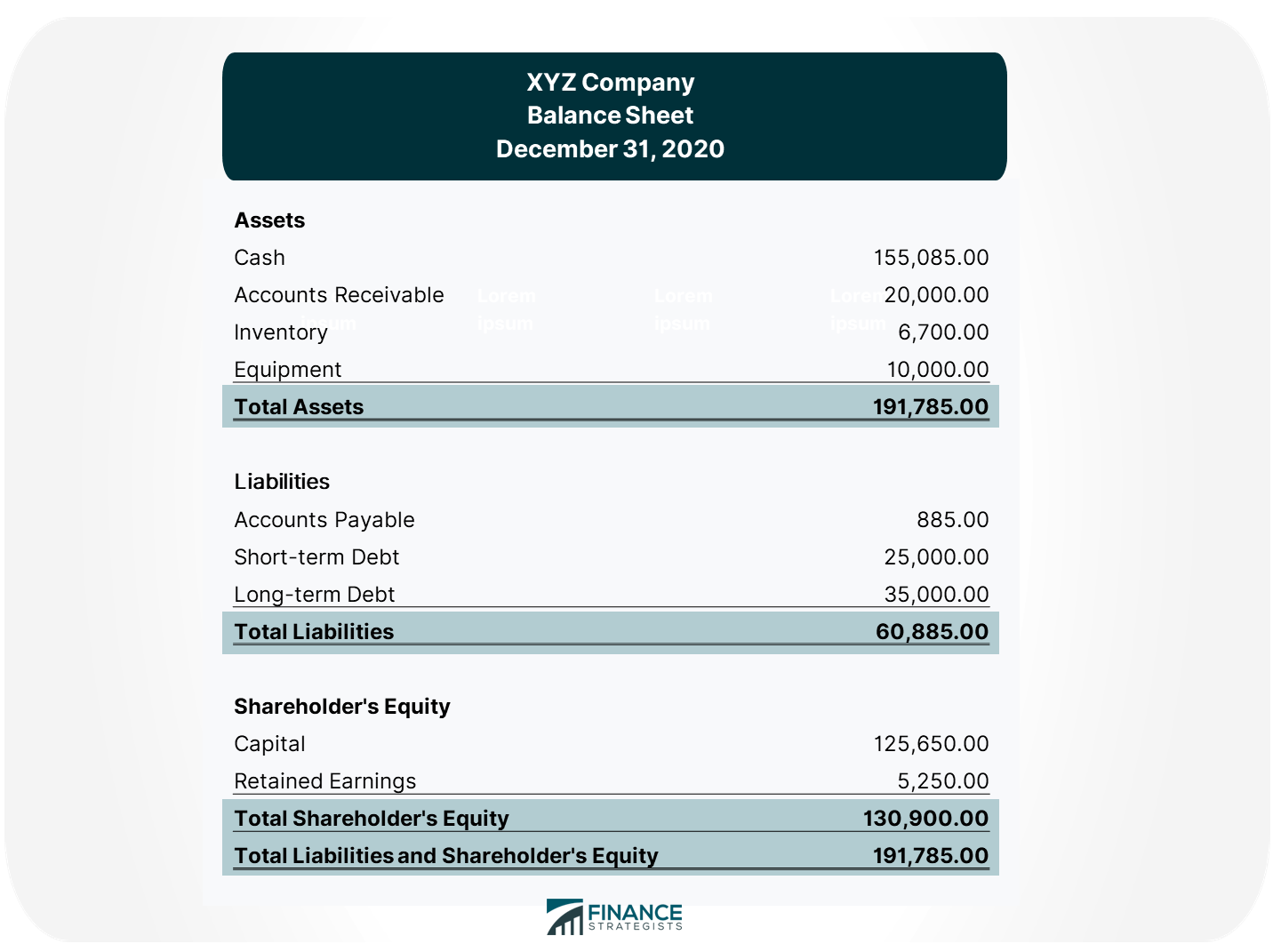# Debt-to-Total-Assets Ratio### Written byTrue Tamplin, BSc, CEPF® | Reviewed by Editorial Team

Updated on December 20, 2022

The debt to total assets ratio describes how much of a company's assets are financed through debt. It is also otherwise known as the debt ratio.

This measure is closely watched by lenders and creditors since they want to know whether the company owes more money than it possesses.

## Why Is Debt-To-Total-Assets Ratio Important?

The debt-to-total-assets ratio is important for companies and creditors because it shows how financially stable a company is. A higher debt-to-total-assets ratio indicates that there are higher risks involved because the company will have difficulty repaying creditors.

A company in this case may be more susceptible to bankruptcy if it cannot repay its lenders. Thus, lenders and creditors will charge a higher interest rate on the company's loans in order to compensate for this increase in risk.

Furthermore, prospective investors may be discouraged from investing in a company with a high debt-to-total-assets ratio.

On the other hand, a lower debt-to-total-assets ratio may mean that the company is better off financially and will be able to generate more income on its assets. Higher interest rates can help boost returns for shareholders.

## How to Calculate Debt-To-Total-Assets Ratio

The debt-to-total-assets ratio is calculated by dividing total liabilities by total assets.Total assets may include both current and non-current assets, or certain assets only depending on the discretion of the analyst.

## Example

XYZ Company has recorded the following items in its balance sheet:Calculate the debt-to-total-asset ratio for XYZ Company. This means that 31% of XYZ Company's assets are being funded by debt.

## Understanding the Debt-To-Total-Assets Ratio

Here's how to interpret the results of the debt-to-total-assets ratio:

### Ratio = 1

A ratio that equates to 1 or a 100% debt-to-total-assets ratio means that the company's liabilities are equally the same as with its assets.
The company in this situation is highly leveraged which means that it is more susceptible to bankruptcy if it cannot repay its lenders.

### Ratio < 1

A ratio that is less than 1 or a debt-to-total-assets ratio of less than 100% means that the company has greater assets than liabilities.
This may be advantageous for creditors because they are likely to get their money back if the company defaults on loans.

### Ratio > 1

A ratio that is greater than 1 or a debt-to-total-assets ratio of more than 100% means that the company's liabilities are greater than its assets.
In this case, the company is not as financially stable and will have difficulty repaying creditors if it cannot generate enough income from its assets.

## Final Thoughts

The debt-to-total-assets ratio is a very important measure that can indicate financial stability and solvency. This ratio shows the proportion of company assets that are financed by creditors through loans, mortgages, and other forms of debt. While it may be beneficial for companies to have lower debt ratios in order to attract investors, this number should not be too low because the company will need some level of funding in order to operate successfully.

## Debt-to-Total-Assets Ratio FAQs

### What is the debt-to-total-assets ratio used for?

The debt-to-total-assets ratio is a popular measure that looks at how much a company owes in relation to its assets. The results of this measure are looked at by creditors and investors who want to know how financially stable a company can be.

### How does the debt-to-total-assets ratio differ from other financial stability ratios?

Other common financial stability ratios include times interest earned, days sales outstanding, inventory turnover, etc. These measures take into account different figures from the balance sheet other than just total assets and liabilities.

### What is the formula for the debt-to-total-assets ratio?

Debt/Asset Ratio = Total Liabilities / Total Assets Where: Total Liabilities = Short-Term Debt + Long-Term Debt Total Assets = Current Assets + Non-Current Assets (or only certain assets) The debt to total assets ratio can be calculated by dividing a company's short and long-term debts by its total assets. This gives an indicator of how much investment or finance has been used in order to fund a company's operations. The lower this figure, the better as it means that the company has been less reliant on external funding from financial institutions and equity investors.

### What if liabilities are greater than assets?

This indicates that the company has negative equity, also known as a "negative net worth." This means that it would be more difficult for the company to borrow money from creditors because they may not see enough value in its assets compared with what is owed.

### Why does the debt-to-total-assets ratio change over time?

The debt-to-total-asset ratio changes over time based on changes in either liabilities or assets. If there is a significant increase in total liabilities, then this will affect the debt-to-total asset ratio positively. Similarly, a decrease in total liabilities leads to a lower debt-to-total asset ratio. On the other hand, a change in total assets will lead to a change in the debt-to-total asset ratio in the opposite direction, either positive or negative.